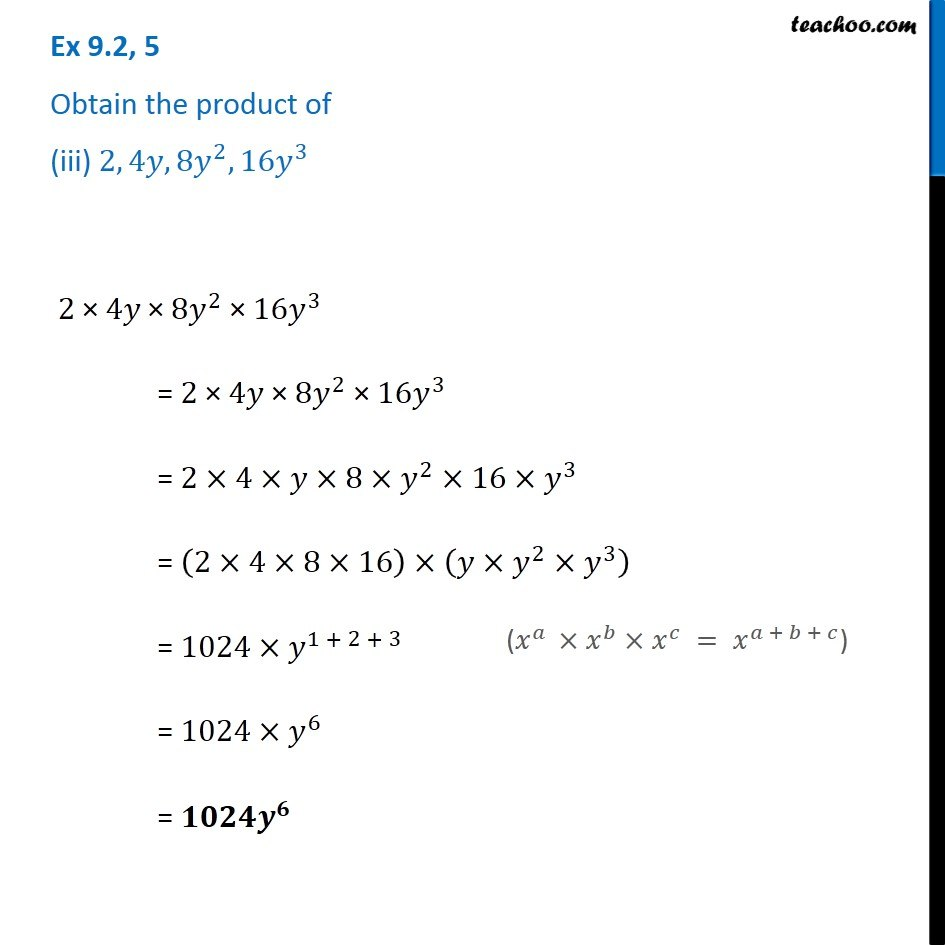Ex 9.2

Chapter 9 Class 8 Algebraic Expressions and Identities
Serial order wiseGet live Maths 1-on-1 Classs - Class 6 to 12

### Transcript

Ex 9.2, 5 Obtain the product of (iii) 2, 4𝑦, 8𝑦^2, 16𝑦^3 2" ×" 4𝑦" ×" 8𝑦^2 " ×" 16𝑦^3 = 2 × 4𝑦 × 8𝑦^2 × 16𝑦^3 = 2×4×𝑦×8×𝑦^2×16×𝑦^3 = (2×4×8×16)×(𝑦×𝑦^2×𝑦^3 ) = 1024×𝑦^(1 + 2 + 3) = 1024×𝑦^6 = 𝟏𝟎𝟐𝟒𝒚^𝟔 (𝑥^𝑎 ×𝑥^𝑏×𝑥^𝑐 = 𝑥^(𝑎 + 𝑏 + 𝑐))